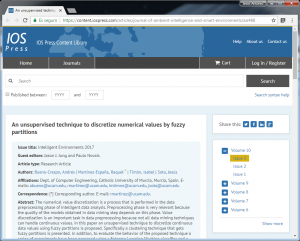# An unsupervised technique to discretize numerical values by fuzzy partitionsUna nueva publicación con técnicas aplicadas al análisis de datos.

### An unsupervised technique to discretize numerical values by fuzzy partitions.

Abstract:

The numerical value discretization is a process that is performed in the data preprocessing phase of intelligent data analysis. Preprocessing phase is very relevant because the quality of the models obtained in data mining step depends on this phase. Value discretization is an important task in data preprocessing because not all data mining techniques can handle continuous values. In this paper an unsupervised technique to discretize continuous data values using fuzzy partitions is proposed. Specifically a clustering technique that gets fuzzy partitions is presented. In addition, to evaluate the behavior of the proposed technique a series of experiments have been proposed using a Extreme Learning Machine classifier and a committee of Extreme Learning Machine. Beside comparing with the K-means discretization technique. These experiments have been validated statistically obtaining the best results the approach proposed.